# Add comparative and cumulative date/time calculations in Amazon QuickSight

Amazon QuickSight recently added native support for comparative (e.g., year-over-year) and cumulative (e.g., year-to-date) period functions which allow you to easily introduce these calculations in business reporting, trend analysis and time series analysis. This allows authors in QuickSight to implement advanced calculations without having to use complicated date offsets in calculations to achieve such datetime-aware comparisons.

In this post, we introduce the new period functions and their capabilities, and demonstrate several typical use cases. We also discuss several scenarios to extend the usage of the period functions, which will be useful in more advanced situations.

## New period functions

Before we demonstrate use cases, let’s go over the new period function suite and see what new functions we now support. We can divide period functions into two main groups: comparative (period over period) functions and cumulative (period to date) functions.

### Comparative (period over period) functions

You can use period over period functions to compare measures at different time periods, such as year, quarter, and month. For example, you can compute a year-over-year increase in sales, or week-over-week percentage revenue changes.

A typical comparative period function has the syntax `periodOverPeriodDifference(measure, date, period, offset)`, with two optional arguments: `period` and `offset`.

You can use the `period` argument in the function to define the period granularity of the calculation. The granularity of `YEAR` means year-over-year computation, `Quarter` means quarter-over-quarter, and so on. If the period argument is left empty, the calculation changes based on the period granularity that is chosen (in the field well) to be displayed in the visual.

You can also use the `offset` argument to specify how many periods apart you want to compute the comparison. For instance, a period of a quarter with an offset of `2` means comparing against the previous two quarters.

Note that `period` and `offset` have to be both specified or both left empty. You can’t specify just one of them.

The following table summarizes the three available period over period functions.

 Function Name Function Type Description periodOverPeriodDifference Table Calculation Calculates the difference of a measure over two different time periods as specified by period granularity and offset. periodOverPeriodLastValue Table Calculation Calculates the last (previous) value of a measure from a previous time period as specified by period granularity and offset. periodOverPeriodPercentDifference Table Calculation Calculates the percent difference of a measure over two different time periods as specified by period granularity and offset.

### Cumulative (period to date) functions

You can use period to date functions to calculate metrics within a given period-to-date window. There are two main types of cumulative functions.

• “OverTime” functions, which are table calculations and return outputs for each row in the visual.

E.g., you can use `periodToDateCountOverTime` with `WEEK` granularity to compute a series of week-to-date new customer counts to track the fluctuation of customer engagement.

• Aggregation functions, which output an aggregated value for a fixed period-to-date time range.

E.g., `periodtoDateSum` with `YEAR` granularity returns a single value for the total of the metric from the beginning of the year to the `endDate` provided in the formula. If left blank, the default `endDate` takes `now()`, which is the moment when users load the dashboard.

The following table summarizes the period to date functions.

 Function Name Function Type Description periodToDateAvgOverTime Table Calculation Calculates the average of a measure for a given time granularity (for instance, a quarter) up to a point in time. periodToDateCountOverTime Table Calculation Calculates the count of a dimension or measure for a given time granularity (for instance, a quarter) up to a point in time. periodToDateMaxOverTime Table Calculation Calculates the maximum of a measure or date for a given time granularity (for instance, a quarter) up to a point in time. periodToDateMinOverTime Table Calculation Calculates the minimum of a measure or date for a given time granularity (for instance, a quarter) up to a point in time. periodToDateSumOverTime Table Calculation Calculates the sum of a measure for a given time granularity (for instance, a quarter) up to a point in time. periodToDateAvg Aggregation Averages the set of numbers in the specified measure for a given time granularity (for instance, a quarter) up to a point in time. periodToDateCount Aggregation Calculates the number of values in a dimension or measure for a given time granularity (for instance, a quarter) up to a point in time, including duplicates. periodToDateMax Aggregation Returns the maximum value of the specified measure for a given time granularity (for instance, a quarter) up to a point in time. periodToDateMedian Aggregation Returns the median value of the specified measure for a given time granularity (for instance, a quarter) up to a point in time. periodToDateMin Aggregation Returns the minimum value of the specified measure or date for a given time granularity (for instance, a quarter) up to a point in time. periodToDatePercentile Aggregation Calculates the percentile based on the actual numbers in the measure for a given time granularity (for instance, a quarter) up to a point in time. periodToDatePercentileCont Aggregation Calculates the percentile based on a continuous distribution of the numbers in the measure for a given time granularity (for instance, a quarter) up to a point in time. periodToDateStDev Aggregation Calculates the standard deviation of the set of numbers in the specified measure for a given time granularity (for instance, a quarter) up to a point in time based on a sample. periodToDateStDevP Aggregation Calculates the population standard deviation of the set of numbers in the specified measure for a given time granularity (for instance, a quarter) up to a point in time based on a sample. periodtoDateSum Aggregation Adds the set of numbers in the specified measure for a given time granularity (for instance, a quarter) up to a point in time. periodToDateVar Aggregation Calculates the sample variance of the set of numbers in the specified measure for a given time granularity (for instance, a quarter) up to a point in time. periodToDateVarP Aggregation Calculates the population variance of the set of numbers in the specified measure for a given time granularity (for instance, a quarter) up to a point in time.

## Use case 1: Using a period over period function to analyze sales growth in fixed and dynamic granularity

Let’s dive into how period over period functions can support typical business and financial use cases. The following example uses periodOverPeriodDifference to calculate YoY sales growth. Because we have `Segment` and `Region` in the visual, the YoY sales is calculated for each segment and region.

We define the measure of `YoYSales` with the following formula: `YoYSales=periodOverPeriodDifference(sum(Sales),{Order Date},YEAR,1)`

The first argument, `sum(Sales)`, tells the function to calculate based on this measure. The second argument, `Order Date`, specifies the date/time column from which `Year` information is extracted. The third argument, `YEAR`, fixes the granularity of this calculation. When this optional argument is specified, this measure always returns YoY (not QoQ or MoM) no matter how `Order Date` is selected (in the field well) to be displayed in the visual. The fourth argument, `1`, specifies the offset of the comparison. In this example, it means we want to compare the sales of each order date with the same date of the previous year. The measure returns empty for order dates of 2018, because no previous periods exist to be compared with.

The period functions are working with totals and subtotals. By adding the total for columns into the visual, you can see the total sales and total `YoYSales` for each region.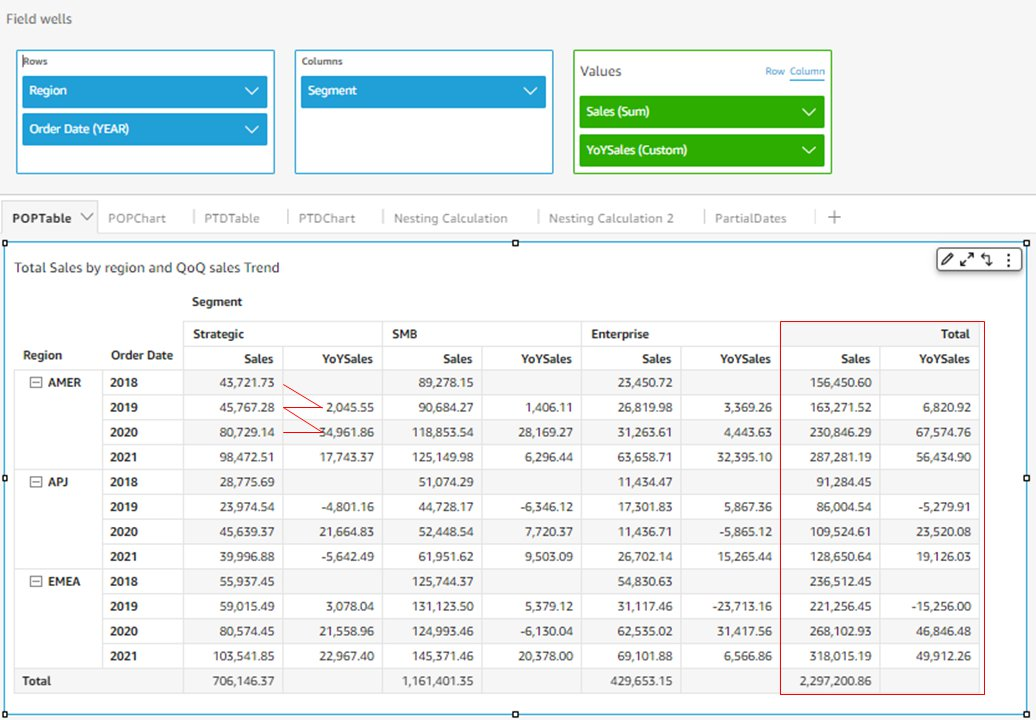If you leave the optional argument of `period` granularity empty, meaning change the formula to `PoPSales=periodOverPeriodDifference(sum(Sales),{Order Date})`as shown in the following example, the time period of the calculation is then determined by the granularity of `Order Date` displayed on the visual. In the following example, Order Date is chosen to display at quarter level (in the field well), so `PoPSales` dynamically calculates the QoQ sales growth. Changing `Order Date` to the monthly level updates the measure to calculate MoM. For `PoPSales`, only Q1 2018 returns empty because that’s the only quarter that doesn’t have a previous quarter to compare with.

If we add `YoYSales` from the previous example to this visual, it calculates YoY sales growth at the quarter level (compares sales of Q1 2019 with Q1 2018). This demonstrates the difference between a fixed granularity and a dynamic granularity of period over period functions.

The period over period functions can differentiate between a positive change (increase) and negative change (decrease). Therefore, when we add the conditional formatting to the visual, it’s very straightforward to see the financial performance of each period (green is good, red is bad).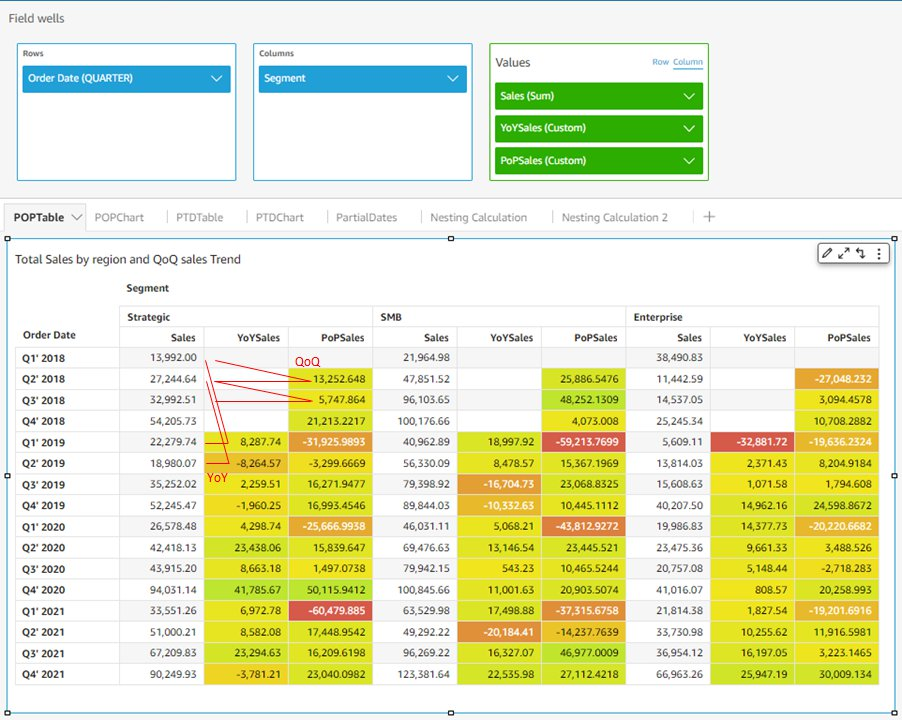Similarly, you can use `periodOverPeriodPercentDifference` to calculate relative sales growth over time. You can add dimensions into the visual to dive further into business insights, such as analyzing the breakdown of each business segment’s sales change by quarter, and their contribution to the total sales increase. We use the formula `PoPSales%=periodOverPeriodPercentDifference(sum(Sales),{Order Date})`.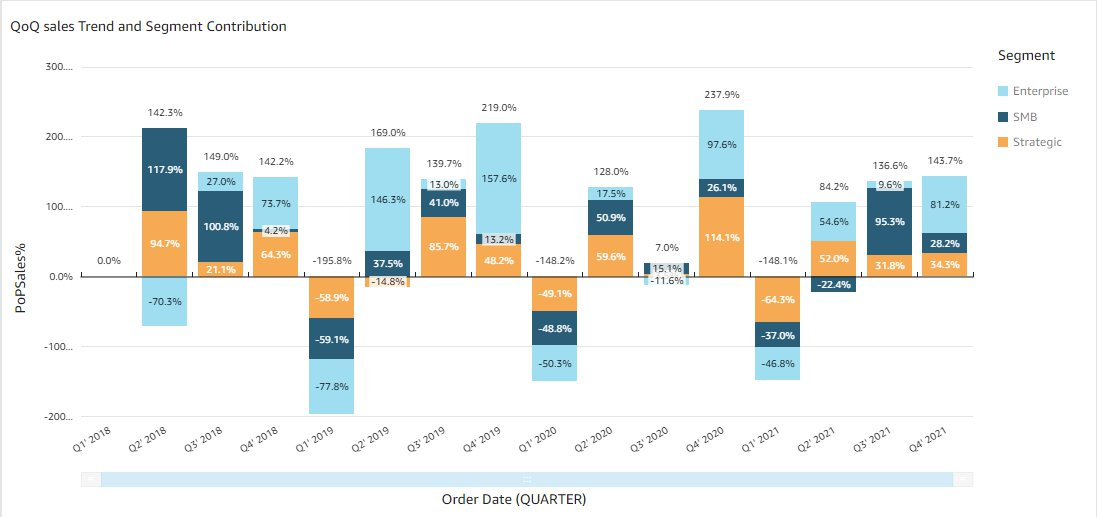## Use case 2: Using a period to date function to track YTD sales in table calculations and aggregations

Similar to period over period functions, the period to date function suite provides a quick and easy way to calculate year-to-date (YTD) or quarter-to-date (QTD) metrics. In the following example, we use the formula of `YTDSumoverSales=periodToDateSumOverTime(sum(Sales),{Order Date},YEAR)`, and `YTDtotalcountSales=periodToDateSumOverTime(count(Sales),{Order Date},YEAR)` to calculate YTD sales and YTD total number of transactions.

Contrary to period over period functions, the third argument of period to date functions, `period`, isn’t optional. Therefore, the calculation granularity is always fixed. In this example, with the granularity defined as YEAR, this measure always calculates YTD, instead of QTD or MTD. Because `Order Date` is displayed at the monthly level, this calculation outputs the YTD sales of each month, and starts over again in January for the next year. As shown in the result table, `YTDSumoverSales` of January 2018 is the monthly sales of January 2018, and `YTDSumoverSales` of February 2018 is the monthly sales of January 2018 plus that of February 2018. And `YTDSumoverSales` of January 2019 goes back to the monthly sales of January 2019.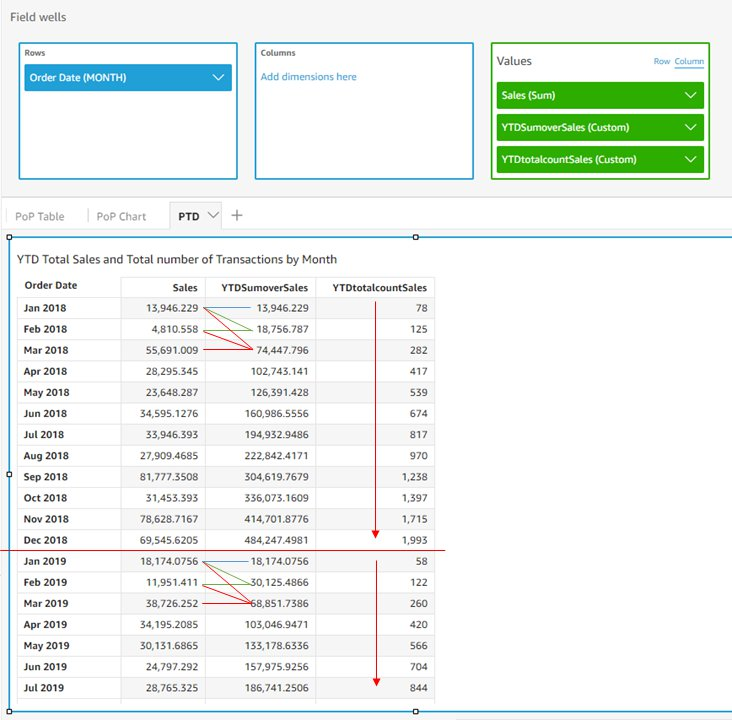You can further dive into the details by populating the calculations in a line chart, and adding more dimensions into the analysis. The following example shows the YTD weekly sales growth trend for each region along the past four years, and uncovers some interesting sales competition between AMER and EMEA in year 2021.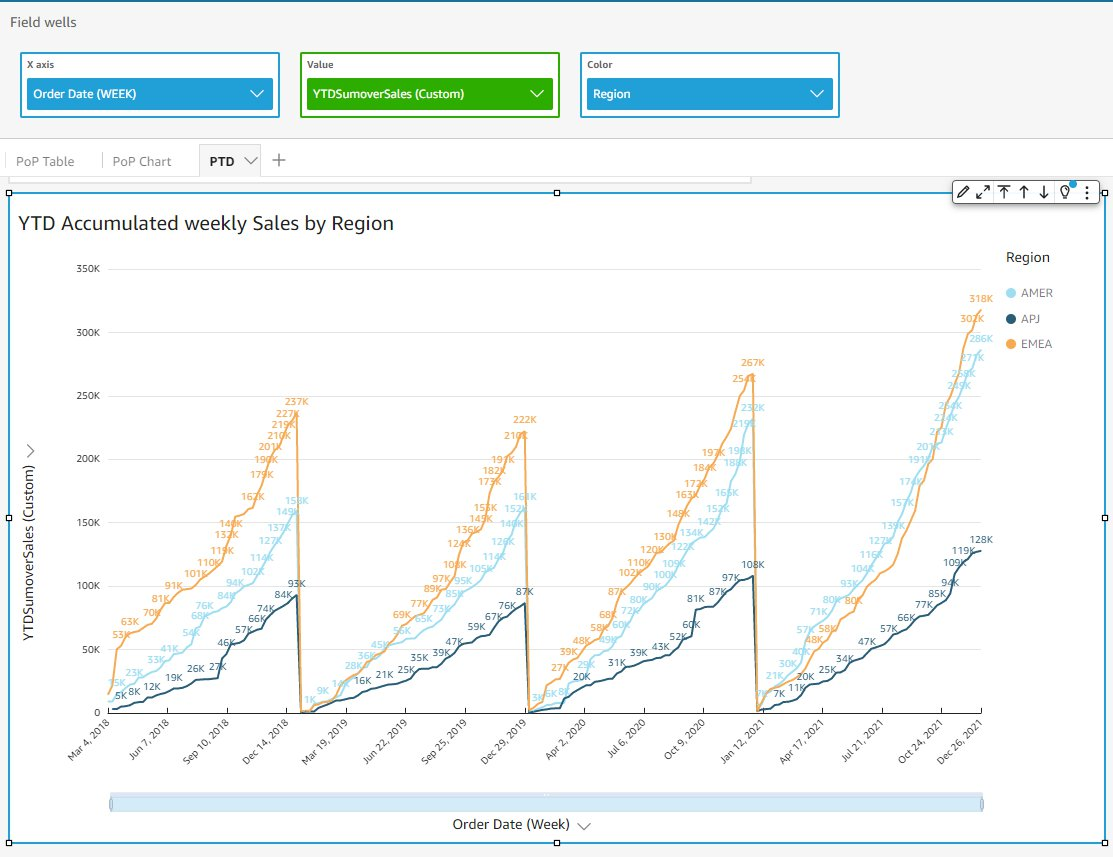In addition to the table calculations, the aggregation period functions are particularly useful when you need to build KPI charts to evaluate YTD metrics in a real-time manner. In the following example, we use the aggregation period to date functions to build two KPI charts to track the YTD total sales, and YTD total number of transactions. For the date December 26, 2021, the timestamp results match the corresponding table calculations for the date of December 26, 2021 in the table. The following table summarizes the formulas.

 Formula Formula Type `YTDSumoverSales=periodToDateSumOverTime(sum(Sales),{Order Date},YEAR)` Table Calculation `YTDtotalcountSales=periodToDateSumOverTime(count(Sales),{Order Date},YEAR)` Table Calculation `YTDSumSales=periodToDateSum(Sales,{Order Date},YEAR)` Aggregation (KPI chart) `YTDCountSales=periodToDateCount(Sales,{Order Date},YEAR)` Aggregation (KPI chart)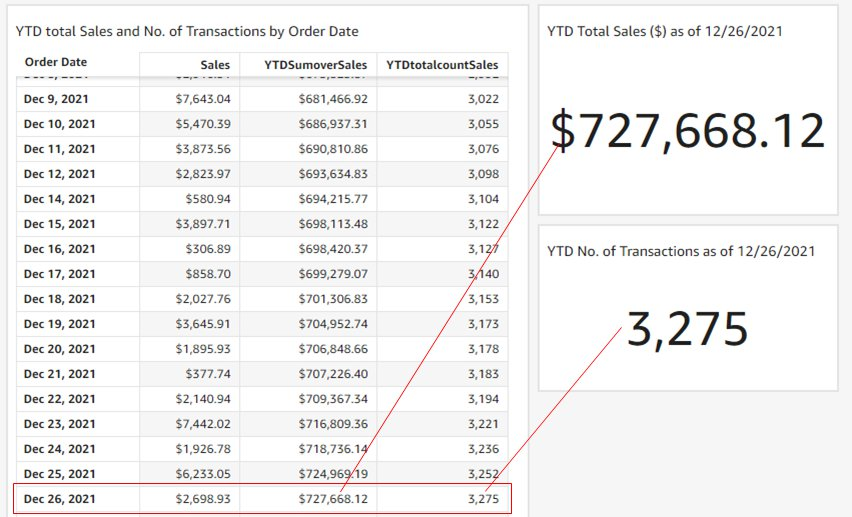## Advanced use case 1: Date/time awareness with period functions

Period functions are not only easier to define and read, they’re also date/time-aware, meaning the functions are calculated based on a date/time-based offset instead of a fixed number of rows. It can solve two major problems that were not possible to be addressed before.

### Period functions can handle varying period duration

If you want to calculate the daily MoM sales increase, you can’t use a fixed offset on each month because the number of days of each month are different (31 days for January and 28 or 29 days for February).

Period functions are calculated based on calendar dates instead of a fixed offset. In the following example, we use the formula `MoMsalesDiff=periodOverPeriodDifference(sum(Sales),{Order Date},MONTH,1)`. The daily MoM increase is calculated correctly based on the day of the month. The sales of the first day of the month are compared with the first day of the previous month, and the same applies to all other days. (Visuals are duplicated for demonstration purposes.)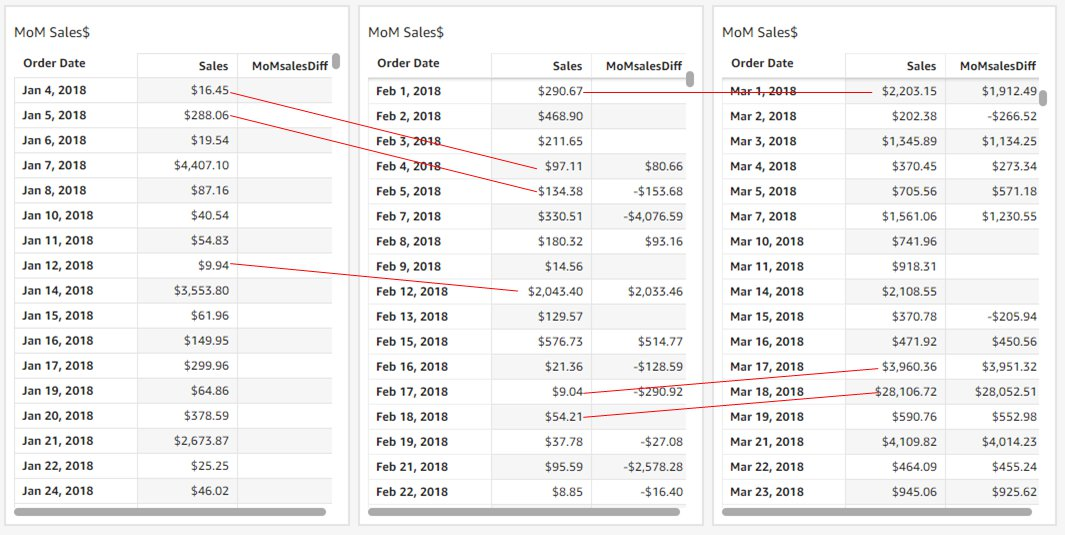### Period functions can handle sparse (missing) data points

Not all datasets can guarantee a complete set of dates. In the previous example, sales data of January 1, 2018, is missing. Using the workaround based on a fixed offset can cause a problem here because we compare February 1, 2018, with a different date instead of January 1, 2018. Period functions always compare measures by date/time offsets so that only desired dates are compared. In the previous example, `MoMsalesDiff` shows empty for February 1, 2018, because of the missing data of January 1, 2018.

## Advanced use case 2: Nesting period functions with other calculations

Now that we can use period over period and period to date functions to create calculated fields, we can nest these functions with other calculations to drive more advanced analysis.

For example, you may want to know for each year, what are the top 10 weeks of the year in regards to week-over-week sales growth. You can do this by calculating `WoWSales` first: `WoWSales=periodOverPeriodDifference(sum(Sales), {Order Date}, WEEK, 1)`. Then you nest it with the `denseRank` window function: `RankWoWSales=denseRank([WoWSales DESC],[{YEAR}])`. This wouldn’t be possible using the fixed-row based workaround, which is implemented using on-visual calculations instead of calculated fields. In the following visual, the top 10 weeks of each year with the highest sales growth are fetched by a simple filter on `RankWoWSales`.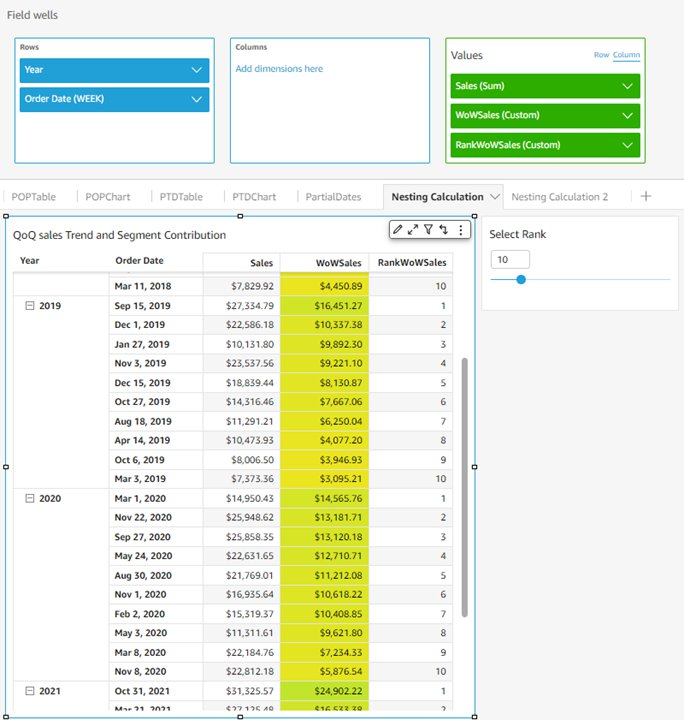You can even nest the period functions with other period functions to generate interesting insights. For example, you can calculate a monthly YoY growth based on the monthly YTD number of transactions. The following formula demonstrates the capability of nesting a YTD calculated field inside a YoY calculated field:

``````YTDtotalsountSales=periodToDateSumOverTime(count(Sales),{Order Date},YEAR)
YoYYTDSalesCount=periodOverPeriodDifference(YTDtotalcountSales,{Order Date},YEAR,1)``````

The results in the following visual show a YoY growth based on a YTD accumulated number of transactions instead of the absolute monthly numbers.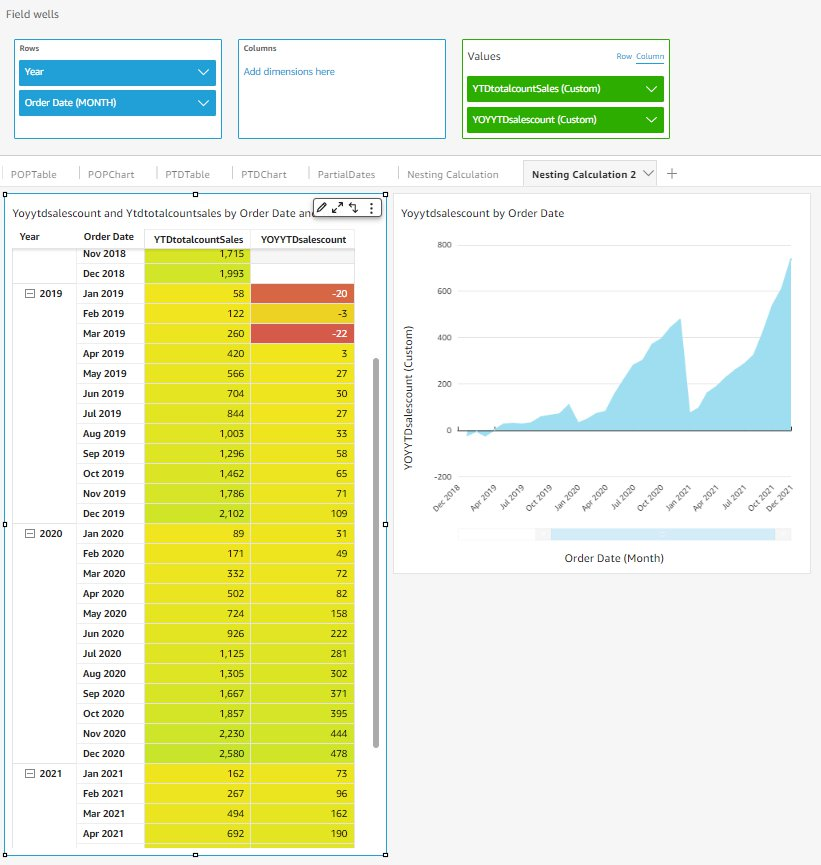## Advanced use case 3: Partial period comparisons

Finally, we discuss a third advanced use case: partial period comparison. Imagine it’s November 15, 2021 (which is the 46th day of the last quarter of 2021), and you want to calculate `4` to compare the performance of this quarter with past quarters, but only using the first 46 days of each quarter instead of the whole quarter. This requires a calculated field using `periodOverPeriodDifference` nested with the `sumIf()` window function.

The following example demonstrates using a nested calculated field to address this use case:

``````QuarterToDate=periodToDateSumOverTime(sum(Sales), {Order Date}, QUARTER)
PartialQTDSales=sumIf(Sales, dateDiff(truncDate("Q", {Order Date}), {Order Date}, "HH") <= dateDiff(truncDate("Q", now()), now(), "HH"))
PartialQoQQTDSales=periodOverPeriodDifference(sumif(Sales, {Order Date} <= addDateTime(dateDiff(truncDate("Q", now()), now(), "HH"), "HH", truncDate("Q", {Order Date}))), {Order Date}, QUARTER, 1)``````

`PartialQTDSales` computes how many hours from the beginning of this quarter to the current date and uses `sumIf()` to calculate the total sales of that period of each quarter. `partialQoQQTDSales` then nests the `periodOverPeriodDifference` function with `PartialQTDSales` to find the partial QoQ differences. Such a comparison based on a partial period isn’t feasible without the new date/time-aware period functions.## Conclusion

In this blog, we introduced new QuickSight period functions which enable quick and powerful date/time-based calculations. We reviewed comparative and cumulative period functions (i.e., period over period and period to date), discussed two major use cases (fixed vs. dynamic granularity and table calculation vs. aggregation), and extended the usage to three advanced use cases. Period functions are now generally available in all supported QuickSight Regions.

Looking forward to your feedback and stories on how you apply these calculations for your business needs.Emily Zhu is a Senior Product Manager at Amazon QuickSight, AWS’s cloud-native, fully managed SaaS BI service. She leads the development of QuickSight core analytics and calculations capability. Before joining AWS, she was working in the Amazon Prime Air drone delivery program and the Boeing company as senior strategist for several years. Emily is passionate about potentials of cloud-based BI solutions and looks forward to helping customers to advance in their data-driven strategy making.Rajkumar Haridoss is a Senior Software Development Engineer for AWS QuickSight. He is the lead engineer on the Query Generation team and works on back-end calculations, query planning, and query generation layer in QuickSight. Outside of work, he likes spending quality time with family and 4-year-old.